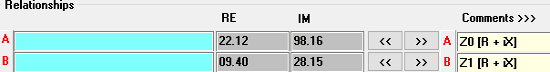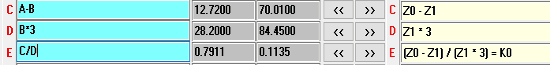Editor Ratings:
User Ratings:
[Total: 6 Average: 5]

OBphasor is a free electrical engineering calculator software for Windows. It lets you calculate electrical equations containing complex numbers. In electrical engineering, for DC current circuits, voltage, resistance, and current are scalar quantities, so their calculation can be done with simple calculators. But when it comes to AC current circuits, voltage, current, and impedance (resistance) have alternate directions and posses other dimensions for amplitude (frequency and phase shift). So in order to represent these multi-dimensional quantities, you need to use complex numbers with real and imaginary parts. Simple calculators cannot carryout calculations of complex numbers. In such situation, Obphasor can come handy, as it can calculate problems with complex numbers for you.

This software directly uses common mathematical operations of “+”, “-“, “*” and “/” with complex quantities. It breaks complex equation into simple steps to do the required calculation. OBphasor lets you use four conversion options: (a, b), (module, angle), (module, cosFi)→(a, b), and L,C→Z. You can also calculate the conjugate for any Input Output (a, b). This helps electrical engineers to calculate electrical equations with real and imaginary parts very easily.Let’s see how this works.

## How to use this Free Electrical Engineering Calculator For Windows:

OBphasor is a portable electrical engineering calculator for Windows, so you don’t really have to install this software. You just have to extract the zip file you have downloaded and run the executable file of OBphasor. On running this software, its interface directly pops out. Here, you need to enter the real and imaginary parts of equations you need to work on.

Let us take a simple example first to understand how this Electrical calculator works:

As you know V=I*Z, where V is voltage, I is current, and Z is impedance.

Lets suppose the Voltage in an AC circuit is 42 + j12 Volts and Impedance is 3.5 + j4.2 Ohms. Then what is the value of Current?

V = I*Z

V = 42 + j12 (volts)→object A

Z= 3.5 + j4.2 (ohm)→object B

I = V/Z (amps)→object C

Fill the values of V and Z in any of the row in RE and IM columns. Real part goes in RE column, while the imaginary part with j goes in IM column. Each equation is represented by the row’s name. Here V is A, while Z is B. Now enter the relationship in C and click RUN. You will get the value of I in real and imaginary part in RE and IM columns of C.I= 6.642 – j4.4965 amps

Now lets take an example of more complex calculation of Earth-Return Compensation Factor (K0) for an Electric Power line:

Suppose you know the value of both the impedance, positive sequence (Z1) and zero sequence (Z0) for an electric power line:

`Z0 = 22.12 + j98.16 (Ohm)→object A`

`Z1 = 09.40 + j28.15 (Ohm)→object B`

Fill the values of Z0 and Z1 as in the first example.We need to calculate K0, that is Earth return compensation factor: K0 = (Z0 – Z1) / (Z1 * 3).

Since OBphasor can only use one mathematical operation at a time, you will need to have patience and solve the above equation in steps. Now we divide the above equation for K0 in following 3 parts.

• `Z0 - Z1 = A-B → object C`
• `Z1 * 3 = B*3 b →object D`
• `K0 = (Z0 - Z1) / (Z1 * 3) = C/D →object E`

Now, enter the relationship in the columns one by one. Enter the relationship for row C, then click on Run. Do the same for next 2 steps to reach the final value of K0. So simple, isn’t it?The phasor form of K0 obtained is 0.7911 – j0.1135.

In order to convert this phasor form of K0 (a,b) to (module, angle), you need to paste the value of K0 to input output panel, then click on (module, angle) option. And, this gives you the value of K0 in module and angle.

`K0 = 0.7992 /_8.1646 deg.`There are two more conversion option available with OBphasor named: (module, cosFi)→(a,b) and L,C→Z.

OBphasor also has the option to save a calculation as .mc file on computer. There is also an option to save the calculation as a PDF file for printing purpose. On using this option, the screenshot of OBphasor’s interface with the calculations gets saved as a PDF file. There is an option to conjugate input output for (a, b).

## Verdict:

This is a great desktop electrical engineering calculator software for electrical engineers. It does well with its job to calculate complex numbers with real and imaginary parts. The process to use OBphasor is a bit slow, as it performs one action at a time. But on the bright side, it lets you save whole calculation for future references. I will recommend this to every electrical engineer.

Get it from here.